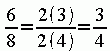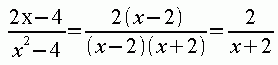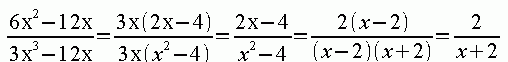SEARCH HOMEMath Central Quandaries & QueriesQuestion from TAMIKA, a student: X^2 + 10X + 24 ______________ X + 6 SIMPLIFY EACH RATIONAL EXPRESSION (IF YOU CAN'T SIMPLIFY IT, WRITE ALREADY SIMPLIFIED)Hi Tamika.

When you are asked to simplify a fraction, you look for a common factor (something that goes into the top and the bottom). Then you can cancel out that factor to simplify it. Here is a simple example:As you see, I noticed that 2 goes into both the top and the bottom, so I factored it out, cancelled them and am left with the final answer.

With algebraic expressions, it works the same way. Here is another example:Above, I factored the top and the bottom, saw a common factor of (x-2) and so I cancelled that factor to simplify it.

Your question is solved exactly the same way. First, factor the top and the bottom. Next, cancel the common factor from the top and bottom to find your answer.

With some questions, you might need to do this more than once. Here is an example (the question you sent isn't nearly as complicated as this, though):